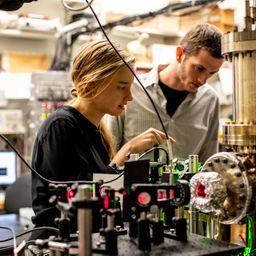## 2.00 \mathrm{eV}

Quantum Physics

Atomic Physics

### Discussion

You must be signed in to discuss.
##### Top Physics 103 Educators##### Andy C.

University of Michigan - Ann Arbor##### Aspen F.

University of Sheffield##### Jared E.

University of Winnipeg### Video Transcript

Okay, so this problem were given information about two different light sources hitting a metal. And we want to get the work function of the metal. Um, and so let's go ahead and write down the givens. So, um, lambda, uh, just playing lambda corresponds to a kinetic energy. Um, of one equals 1.0 e b. And then Lambda over, too. Gives a chaotic energy bill. Is it JJ for you mean you want to get the work function of the metal so we won't fi. And so let's go ahead and do the algebra that we needed. So, in general, the formula relates connect energy to a work function as kinetic energy is HC over lambda minus the work function. And so, um, yeah, so we can kind of write this down. And in either case, so we can say for the 1st 1 you can say that I'm gonna actually just kind of play. Do you like a mixture of numbers And, um, okay. And variables. Sometimes I think it's easier I'll do it if I don't have to create new symbols. I guess I'm already created Katie. Want Okay too. Um, you know what I'm gonna do it. So So we have one. Is HC divided by Lambda minus Phi and then for equals Tau Lambda over to. So that's two a C over Lambda minus phi. So what we can do is we can solve, um, one of these for fei and plug it into here and just kind of to equation to unknown. You know, I really changed my mind. I was so gung, How about mixing variables and numbers? But now that I've done it, I feel uncomfortable. Like they're all kind, different units. It probably really doesn't matter. There we go. Now I feel a little more comforted. Great. And so now we want to do is yes. Let's take this in sulfur lambda. So I'm gonna bring this fight the other side kind of working, right, So left s okay. Plus five is equal to age. See over Lambda. And then, um, you can cross, multiply or whatever you want to call it to get Lambda Sage, see divided by K E. Plus five key one spy. And then then you can go ahead and put this in here. Let's go and solve this Verify. So we got five is equal to, um to age see over Lambda. And I'm just so I'm solving fry than plugging in This slammed us So we're gonna take this H c divided by K one. It was Oh, goodness. This is sort of uses to solve this for fi. That's okay. This agency's gonna cancel, so that will be fine. Um, Okay. Great. And so now, Smith, this to little more upstanding, respectable. Great. And so now what we can do is we can cancel the age, see? And then we can move this up to the numerator, so I'm just gonna work right to left. So then we get five is equal Thio to K e t. One plus fi minus Katie, too. Oh, yes, it's not bad. So then you could bring this to fight to the other side and then divide the whole thing by two. Um, so I'm just gonna kind of I guess I guess I could use this section. So So it's kind of kind of be a mess. You go ahead and, like, put some little blocks on things. So here is the kind of the first part, and I got this funny progression of moving right to left. And then this is gonna you gonna finish up over here? So this is kind of just algebra. And so yeah, so then I'm gonna have too. So have to fi on this side one. Find the other side. So one of the buyers will cancel on this size. Will have a fine this site. So you just bring everything else over to the other side. So I got five is equal to two k e go, um, one negative to Katie. One plus que tu. And now I can go have employment numbers. So Katie two is four minus two times one is two. So that's gonna be 2.0 electron rules.University of Washington

#### Topics

Quantum Physics

Atomic Physics

##### Top Physics 103 Educators##### Andy C.

University of Michigan - Ann Arbor##### Aspen F.

University of Sheffield##### Jared E.

University of Winnipeg